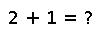# isprime(::BigInt, ?)

isprime(x::BigInt, [reps = 25]) -> Bool

Probabilistic primality test. Returns `true` if `x` is prime; and `false` if `x` is not prime with high probability. The false positive rate is about `0.25^reps`. `reps = 25` is considered safe for cryptographic applications (Knuth, Seminumerical Algorithms).

``````julia> isprime(big(3))
true``````

## Examples

Checking you are not a robot: